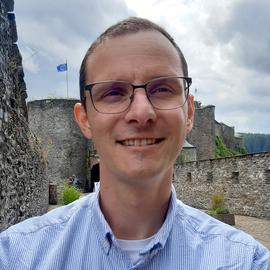# Dithered quantized compressive sensing with arbitrary RIP matrices

Date
Nov 1, 2018
Location
Banff International Research Station, Canada

Abstract: Quantized compressive sensing (QCS) deals with the problem of coding compressive measurements of low-complexity signals (e.g., sparse vectors in a given basis, low-rank matrices) with quantized, finite precision representations, i.e., a mandatory process involved in any practical sensing model. While the resolution of this quantization clearly impacts the quality of signal reconstruction, there also exist incompatible combinations of quantization functions and sensing matrices that proscribe arbitrarily low reconstruction error when the number of measurements increases. In this talk, we will see that a large class of random matrix constructions, i.e., known to respect the restricted isometry property (RIP) in the compressive sensing literature, can be made “compatible” with a simple scalar and uniform quantizer (e.g., a rescaled rounding operation). This compatibility is simply ensured by the addition of a uniform random vector, or random “dither”, to the compressive signal measurements before quantization. As a result, for these sensing matrices, there exists (at least) one method, the projected back projection (PBP), capable to estimate low-complexity signals from their quantized compressive measurements with an error decaying when the number of measurements increases. Our analysis is developed around a new property satisfied with high probability by the dithered quantized random mappings, i.e., the limited projection distortion (LPD), which enables both uniform and non-uniform (or fixed signal) reconstruction error guarantees for PBP.